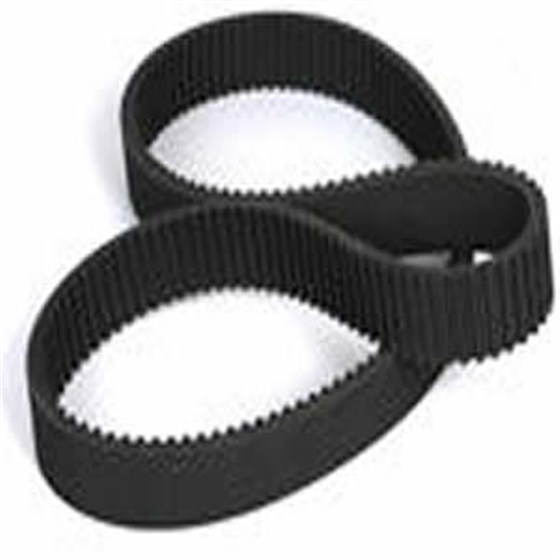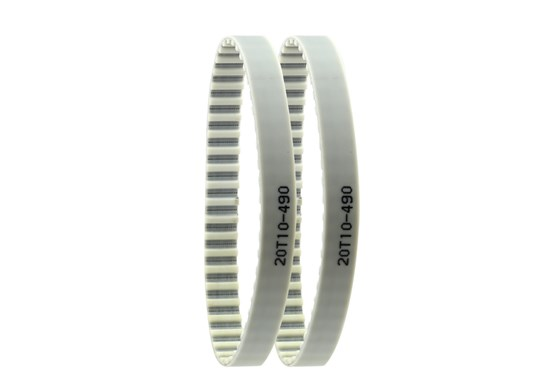﻿ 如何设计同步带_深圳市合发齿轮机械有限公司

# 如何设计同步带## 同步带的设计计算：

1、对于电机额定输出功率的估算

P=Tw=4.8×0.1÷0.067=7.16W

2、对于确定计算功率的计算

Pca=KaP=7.16×1.7=12.17W

3、对于小带轮转速的计算

Nn=v/r=0.1÷0.067×60=8.96r/min

4、对于小带轮节圆直径的确定

D1=Z1Pb/π=41×12.7÷3.14=165.82mm

5、对于大带轮相关数据的确定

6、对于带速v的确定

V=πdn÷(60×1000）=(3.14×117.29×8.96)÷(60×1000)=0.1m/s≦Vmax